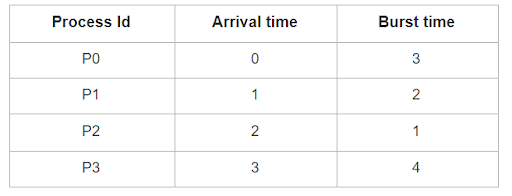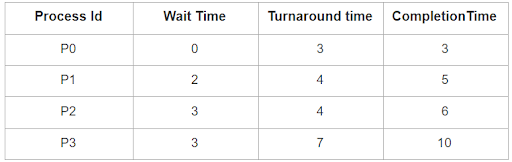# FCFS Scheduling Algorithm with Different Arrival Time

Posted: 7 Apr, 2021
Difficulty: Moderate

## PROBLEM STATEMENT

#### Note:

``````1. All the processes will have different arrival times.

2. In the FCFS scheduling algorithm, processes that come first ( i.e have lesser arrival time) will be executed first.

3. Turn around time of a Process is the time difference between arrival time and the time at which the process completed.

4. The waiting time of a process is the total time, the process is waiting, i.e. difference between turnaround time and burst time.

5. Completion time is the time at which a process completes.
``````

#### Example:

``````Let there be 4 Processes with the following arrival time and burst time.
````````````Wait time, turnaround, and completion time for each process are:
``````#### Input format:

``````The first line of input contains an integer ‘T’ denoting the number of test cases. The description of ‘T’ test cases follows

The first line of each test case contains an integer ‘N’ representing the number of processes.

The second line of each test case contains ‘N’ space-separated integers, where ‘i-th’ integer represents the arrival time for the ‘i-th’ processes.

The last line of the test cases contains ‘N’ space-separated integers, where ‘i-th’ integer represents the burst time for the ‘i-th’ processes.
``````

#### Output format :

``````For each test case, print four lines.

In the first line, print ‘N’ space-separated integers, where ‘i-th’ integer represents the waiting time of the ‘i-th’ process.

In the second line of each test case, print ‘N’ space-separated integers, where ‘i -th’ integer represents the turnaround time of ‘i-th’ process.

In the third line of each test case, print ‘N’ space-separated integers, where ‘i -th’ integer represents the completion time of ‘i-th’ process.

In the last line print two space-separated integers, representing the floor value of average waiting time and the average turnaround time respectively.

The output of each test case will be printed in a separate line.
``````

#### Note:

``````You do not need to print anything, it has already been taken care of. Just implement the given function.
``````

#### Constraints:

``````1 <= T <= 5
1 <= N <= 5000
0 <= arrival[i], burst[i] <= 10 ^ 9

Where ‘T’ is the total number of test cases, ‘N’ is the number of processes, and ‘arrival[i]’,  ‘burst[i]’ represents the arrival time and burst time of the ‘i-th’ processes.

Time Limit: 1 sec
``````Approach 1

Approach: As the CPU scheduling algorithm is First Come First Serve, So the process with minimum arrival time will be executed first, when this process is completely executed, then only the next process with minimum arrival time will start executing.

Turn around time of a process is the time span from its arrival to its completion. For example, if a process arrives at 5 unit time and it completes at 10 unit time, then its turnaround time will be (10 - 5) units.

The waiting time of a process is the time span from its arrival to the stage when this process starts executing. When a process starts executing immediately then its arrival time will be 0.

To Calculate waiting time and turnaround time for all the processes, we iterate over all processes in ascending order with respect to their arrival time, and then find their start time i.e time when they start executing, completion time i.e. time when the process gets completed. From these, we can easily calculate their turnaround time and wait time by the below formula.

Start time = max (arrival time of current process, completion time of the previous process)

Completion time = start time + burst time of the current process.

Turnaround time =  completion time - arrival time of the current process.

Waiting time = Turnaround time - burst time of the current process.

Finally, we can calculate the average waiting time by summing all waiting times of all given processes and divide it by total process. Similarly, the average turnaround time will be the summation of the turnaround time of all the processes divided by a total number of processes.

Algorithm:

1. Sort the processes in ascending order according to their arrival time.
2. Declare two arrays say ‘WAITT’ and ‘TURNT’ to store wait time and turnaround time for each process.
3. Declare two variables say ‘AVGARRIVAL’  and ‘AVGTURN’ to store average arrival time and average turnaround time
4. Declare a variable say ‘PREVIOUS’ and initialize it to 0. It will keep track of the completion time of the previous process.
5. Run a loop to iterate through each Process.
• Let the current process be ‘CURRPROCESS’.
• Declare two variables ‘STARTT’ and ‘COMPLETIONT’
• Set ‘STARTT’ to max ( ‘CURRPROCESS' -> 'ARRIVALTIME’, ‘PREVIOUS’) because the current process can only start when the previous process ends.
• ‘COMPLETIONT’ = ‘STARTT’ + ‘CURRPROCESS' -> BURSTTIME’
• ‘TURNT[ ‘CURRPROCESS -> ID’ ]’  = ‘COMPLETIONT’ - ‘CURRPROCESS -> ARRIVAL’.
• ‘WAITT [ ‘CURRPROCESS -> ID’ ]’ =  ‘TURNT[ ‘CURRPROCESS -> ID’ ]’ - ‘CURRPROCESS -> BURSTTIME’
• ‘AVGARRIVAL’ += ‘WAITT [ ‘CURRPROCESS -> ID’ ]’
• ‘AVGTIME’ += ‘TURNT[ ‘CURRPROCESS -> ID’ ]’
• Update ‘PREVIOUS’ to ‘COMPLETIONT’.
6. Divide ‘AVGARRIVAL’ and ‘AVGTIME’ with the total number of processes and then return both.